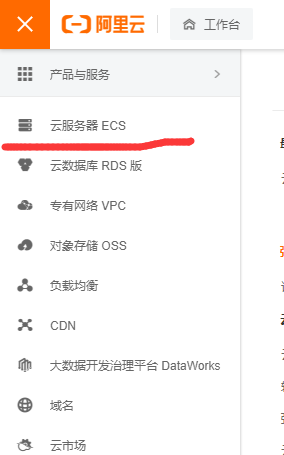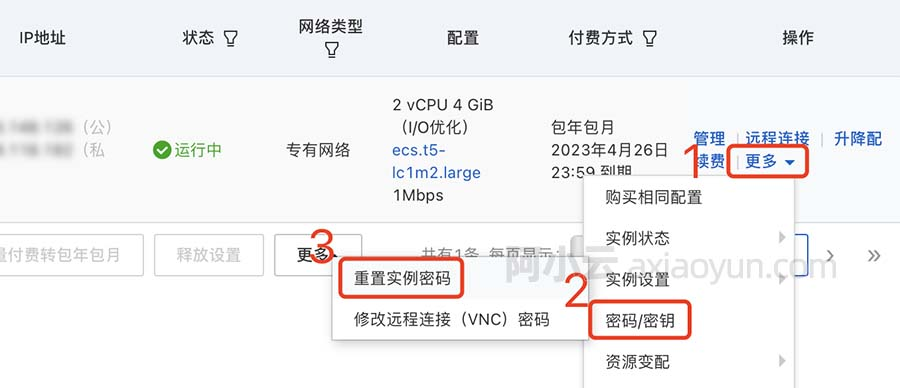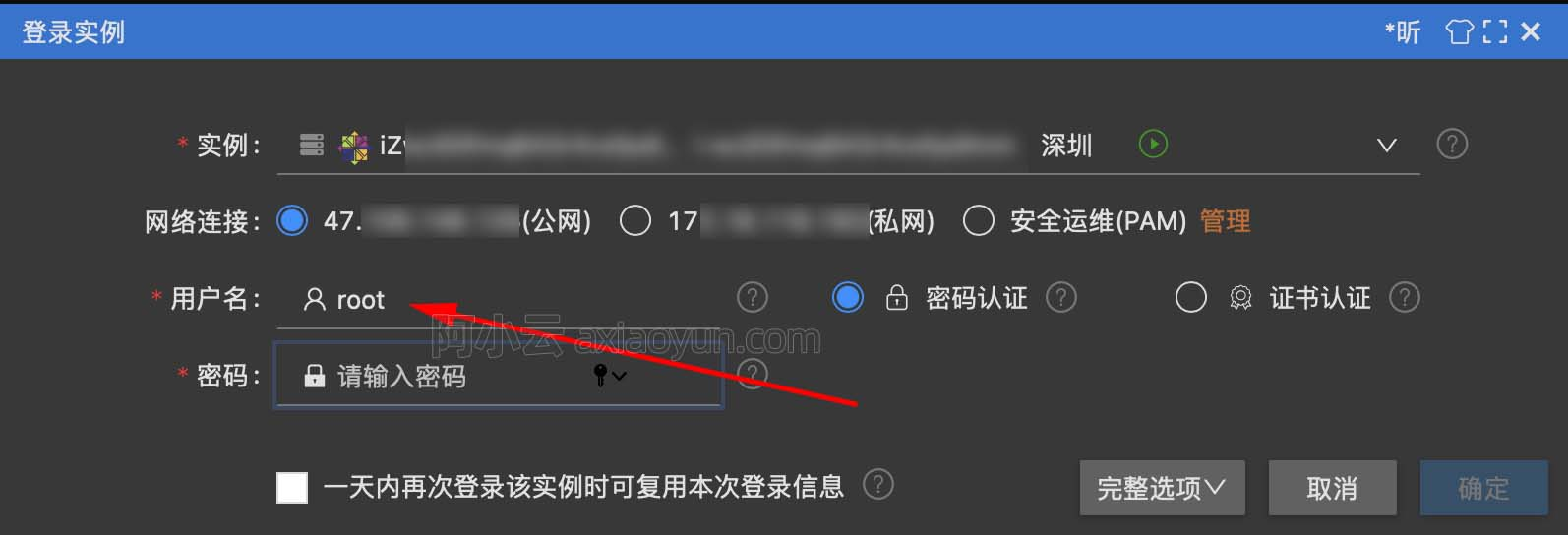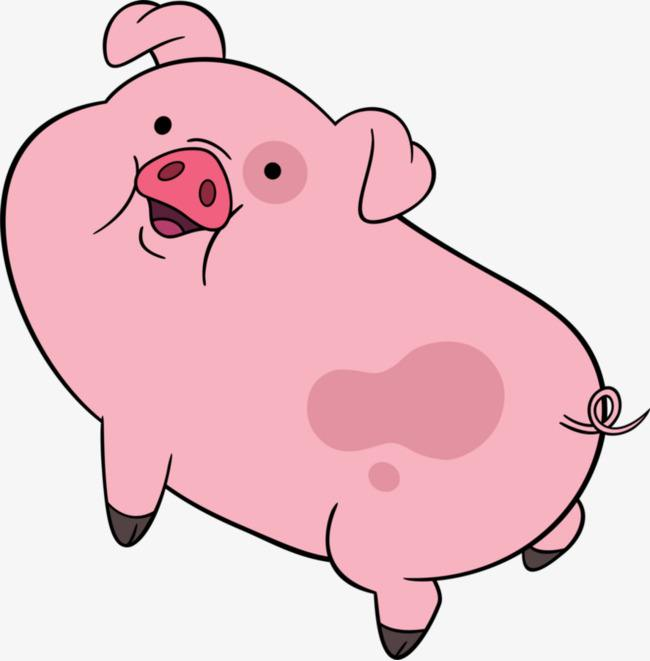# c++ 覆盖、重载、隐藏

+关注继续查看

① double calculate(double);

② double calculate(double，double);

③ double calculate(double, int);

④ double calculate(int, double);

⑤ double calculate(int);

⑥ float calculate(float);

⑦ float calculate(double);

1) 相同的范围（在同一个类中）；

2) 函数名字相同；

3) 参数不同；

4) virtual关键字可有可无。

1) 不同的范围（分别位于派生类与基类）；

2) 函数名字相同；

3) 参数相同；

4) 基类函数必须有virtual关键字。

#include <iostream.h>

class Base

{

public:

void f(int x){ cout << "Base::f(int) " << x << endl; }

void f(float x){ cout << "Base::f(float) " << x << endl; }

virtual void g(void){ cout << "Base::g(void)" << endl;}

};

class Derived : public Base

{

public:

virtual void g(void){ cout << "Derived::g(void)" << endl;}

};

void main(void)

{

Derived d;

Base *pb = &d;

pb->f(42); // 运行结果: Base::f(int) 42

pb->f(3.14f); // 运行结果: Base::f(float) 3.14

pb->g(); // 运行结果: Derived::g(void)

}

1) 如果派生类的函数与基类的函数同名，但是参数不同。此时，不论有无virtual关键字，基类的函数将被隐藏（注意别与重载混淆）。

2) 如果派生类的函数与基类的函数同名，并且参数也相同，但是基类函数没有virtual关键字。此时，基类的函数被隐藏（注意别与覆盖混淆）。

#include <iostream.h>

class Base

{

public:

virtual void f(float x){ cout << "Base::f(float) " << x << endl; }

void g(float x){ cout << "Base::g(float) " << x << endl; }

void h(float x){ cout << "Base::h(float) " << x << endl; }

};

class Derived : public Base

{

public:

virtual void f(float x){ cout << "Derived::f(float) " << x << endl; }

void g(int x){ cout << "Derived::g(int) " << x << endl; }

void h(float x){ cout << "Derived::h(float) " << x << endl; }

};

1) 函数Derived::f(float)覆盖了Base::f(float)。

2) 函数Derived::g(int)隐藏了Base::g(float)，注意，不是重载。

3) 函数Derived::h(float)隐藏了Base::h(float)，而不是覆盖。

void main(void)

{

Derived d;

Base *pb = &d;

Derived *pd = &d;

// Good : behavior depends solely on type of the object

pb->f(3.14f); //运行结果: Derived::f(float) 3.14

pd->f(3.14f); //运行结果: Derived::f(float) 3.14

// Bad : behavior depends on type of the pointer

pb->g(3.14f); //运行结果: Base::g(float) 3.14

pd->g(3.14f); //运行结果: Derived::g(int) 3

// Bad : behavior depends on type of the pointer

pb->h(3.14f); //运行结果: Base::h(float) 3.14

pd->h(3.14f); //运行结果: Derived::h(float) 3.14

}

===================================================================
-------------------------------------------------------------------
-------------------------------------------------------------------

A派生了B

#include <iostream>
using namespace std;
class A
{
public:
virtual void show()
{
cout << a << endl;
}

int a;
};
class B:public A
{
public:
void show()
{
A::show();
//显示的调用自己类中的 "由A类继承过来的show()函数"
//像这种直接显示指出某个类的某个函数时,
//编译器处理方式是这样的: 首先在自己类中找有没有A::show(),如果找到,调用.不在继续在A类中找
//如果找不到,则在显示指出的那个类中(即A类)调用那个函数. 这里当然是在B类中可以找到A::show()
//因为基类中指出了这个函数是virtual函数.
}
int b;
};

int main()
{
A a;
a.a = 3;
a.show();
B b;
b.b = 4;
b.show();
b.A::show(); //显示的调用自己类中的 "由A类继承过来的show()函数"
return 0;
}

virtual, 覆盖.

-----------------------------------------------------------------------------------------------------------------------

1,同名同参无virtual
2,同名不同参不管有无virtual

class A
{
public:
void show() {};  //编号1
void rose(int a) {}   //编号2
};
class B:public A
{
public:
void show() {}; //编号3
void rose(int a, int b) {};  //编号4
};
//

B类中其实有两个show(),两个rose();

//----1----//

A *p = new B;
p->show();
p->rose(3);
p->rose(3,5); //error

//----2----//

B b;
b.show();
b.rose(3,5);
b.rose(4); //error18705 017140 09730 013541 014718 022930 021630 013894 017438 025094 0

916

0

JS零基础入门教程（上册）